﻿ 集对分析在人工智能中的应用与进展
«上一篇文章快速检索 高级检索

 智能系统学报2019, Vol. 14Issue (1): 28-43  DOI: 10.11992/tis.2018030300

### 引用本文JIANG Yunliang, ZHAO Keqin. Application and development of set pair analysis in artificial intelligence: a survey[J]. CAAI Transactions on Intelligent Systems, 2019, 14(1): 28-43. DOI: 10.11992/tis.201803030.### 文章历史

1. 湖州师范学院 信息工程学院，浙江 湖州 313000;
2. 诸暨市联系数学研究所，浙江 诸暨 311800

Application and development of set pair analysis in artificial intelligence: a survey
JIANG Yunliang 1, ZHAO Keqin 21. School of Information Engineering, Huzhou Normal University, Huzhou 313000, China;
2. Institution of Zhuji connection Mathematics, Zhuji 311800, China
Abstract: Artificial intelligence is a national development strategy in China. Set pair analysis (SPA) is a method that organically combines deterministic calculation with uncertainty systematic analysis, and has provided a very significant basic idea for artificial intelligence. Set pair analysis has been widely applied in fields such as artificial intelligence, pattern recognition, uncertainty reasoning, intelligent decision making, knowledge ecology, natural language understanding, expert system, neural network, intelligent engineering, and community division, as well as in the evolution of intelligent social network. In this paper, the principles of SPA and connection number are first outlined, and then the applications of SPA in artificial intelligence are summarized to promote further applications.
Key words: natural intelligence    artificial intelligence    set pair analysis    deterministic calculation    uncertainty analysis

1 集对分析的理论与联系数 1.1 基本原理

“测不准原理”对系统分析的启示在于：当分析进入到系统的微观层次时会遇到部分系统参数不能确定的问题。

1.2 基本理论

1.3 联系数

 $\mu = a + bi$ (1)
 $\mu = a + bi + cj$ (2)
 $\mu = a + bi + cj + dk$ (3)
 $\mu = a + bi + cj + dk + el$ (4)
 $\mu = a + bi + cj + dk + el + \cdots + xy$ (5)
 $\mu = \left[ \begin{array}{l} a{_1} + b{_1}i + c{_1}j + d{_1}l + \cdots \\ a{_2} + b{_2}i + c{_2}j + d{_2}l + \cdots \\ \quad\quad\quad\quad \vdots \\ a{_n} + b{_n}i + c{_n}j + d{_n}l + \cdots \end{array} \right]$ (6)
 $\partial \mu = \partial a + \partial bi + \partial cj + \cdots$ (7)
 ${\partial ^2}\mu = {\partial ^2}a + {\partial ^2}bi + {\partial ^2}cj + \cdots$ (8)
 $\vdots$
 ${\partial ^{n - 1}}\mu = {\partial ^{n - 1}}a + {\partial ^{n - 1}}bi + {\partial ^{n - 1}}cj + \cdots$ (9)

 $\begin{array}{l} {\partial ^2}\mu = {\partial ^{2 + }}\mu + {\partial ^{2 - }}\mu =\displaystyle \frac{{\displaystyle\frac{a}{{a + b}}}}{\frac{a}{{a + b}} + \displaystyle\frac{b}{{b + c}}}} +\displaystyle \frac{\frac{b}{{a + b}}}}{{\displaystyle\frac{b}{{a + b}} + \displaystyle \frac{c}{{b + c}}}}j = \\ \qquad\displaystyle\frac{\frac{a}{{a + b}}}}{\frac{a}{{a + b}} +\displaystyle \frac{b}{{b + c}}}} - \displaystyle\frac{\frac{b}{{a + b}}}}{\frac{b}{{a + b}} + \displaystyle\frac{c}{{b + c}}}} \end{array}$ (10)

2 集对分析在人工智能基础研究中的应用 2.1 智能的定义

2.2 自然智能的科学原理

2.3 人工智能

3 集对分析在模式识别中的应用 3.1 基于集对分析的同异反模式识别技术

1) 基于联系数伴随函数的模式分类

$\mu (N,M) = a + bi + cj$ 是关于模式 $N{\text{、}}M$ 的一个同异反3元联系数，则当该联系数的势函数 ${\rm shi}(\mu ) =\displaystyle\frac{{{a}}}{c} > 1$ $b < a$ 时，判定 $N{\text{、}}M$ 为同一类模式；当势函数 ${\rm shi}(\mu ) =\displaystyle \frac{{{a}}}{c} < 1$ $b < c$ 时，判定 $N{\text{、}}M$ 互为相反类模式；当 ${\rm shi}(\mu ) =\displaystyle \frac{{{a}}}{c}= 1$ $b < c$ 时，判定 $N{\text{、}}M$ 互为既不相同也不相反的中介过渡类模式，必要时对中介过渡类模式作深入一个或多个层次的细化同异反分类，此时需要把同异反3元联系数展开成同异反4元联系数(见式(4))、5元联系数(见式(5))等。此外还有基于联系数偏联系数式(7)~(10)的模式分类，基于联系数邻联系数的模式分类，以及基于联系数相互作用联系数的模式分类等，可以参见文献。

2) 基于同异反距离的模式分类

3.2 基于集对分析的指纹识别

1) 确定各个指纹特征的权系数

2) 选取待识别指纹的特征点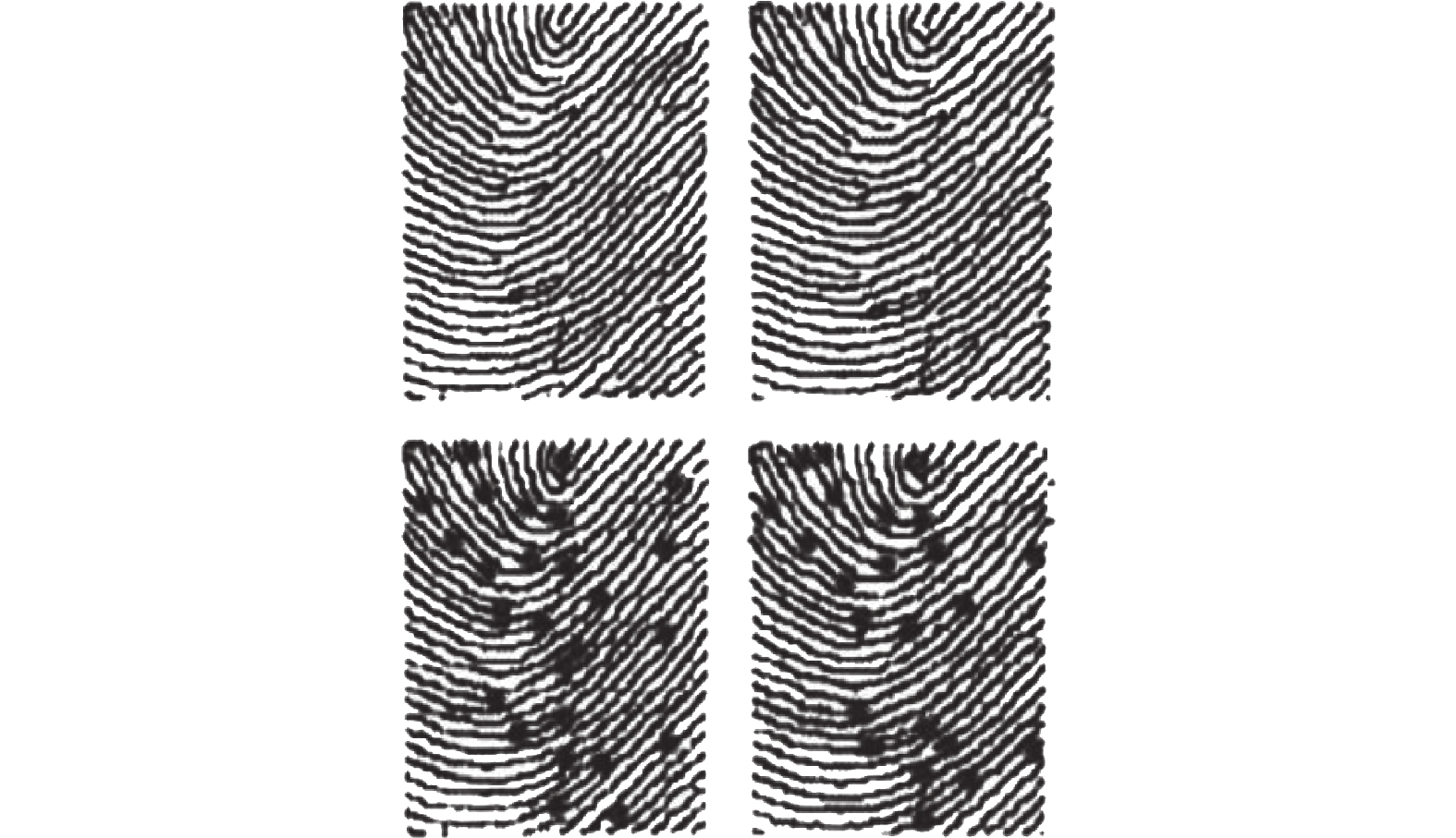Download: 图 1 特征点提取 Fig. 1 Feature point extraction

3) 确定特征点的属性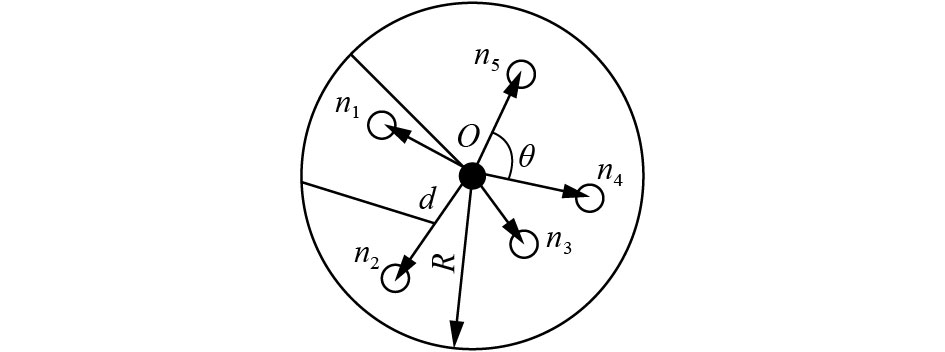Download: 图 2 特征点的属性 Fig. 2 Feature points’ properties

4) 确定各对应特征点对的联系数

 ${\mu _{ijk}} = {a_{ijk}} + {b_{ijk}}i + {c_{ijk}}j$ (11)

 ${a_{ijk}} = {N_a}/N$ (12)
 ${b_{ijk}} = {N_b}/N$ (13)
 ${c_{ijk}} = {N_c}/N$ (14)

5) 指纹图像匹配的确定

 ${R_{ij}} = \left| {{{\left[ {{f_1}\,\,\,\,{f_2}\,\,\,\,\cdots \,\,\,\,{f_m}} \right]}^{\rm{T}}} \times [{\mu _{ij1}}\,\,\,\,{\mu _{ij2}}\,\,\,\,\cdots \,\,\,\,{\mu _{ijm}}]} \right|$ (15)

当 ${R_{ij}}$ 大于某一阈值时，认为待识指纹特征点 $i$ 和模板指纹特征点 $j$ 相同。又当这种相同的点对占两枚指纹图像特征点总量一定百分比时，系统判断这两枚指纹匹配成功。实验证实了成科扬给出的基于集对分析的指纹识别算法在达到99.78%的正确识别率时拒识率仅为0.08%，且在指纹图像有部分缺损、拉伸时，仍具有很好的鲁棒性。

3.3 基于集对分析的其他识别举例

4 集对分析在不确定性推理中的应用 4.1 基于赵森烽−克勤概率的不确定性推理

4.2 基于集对分析的同异反推理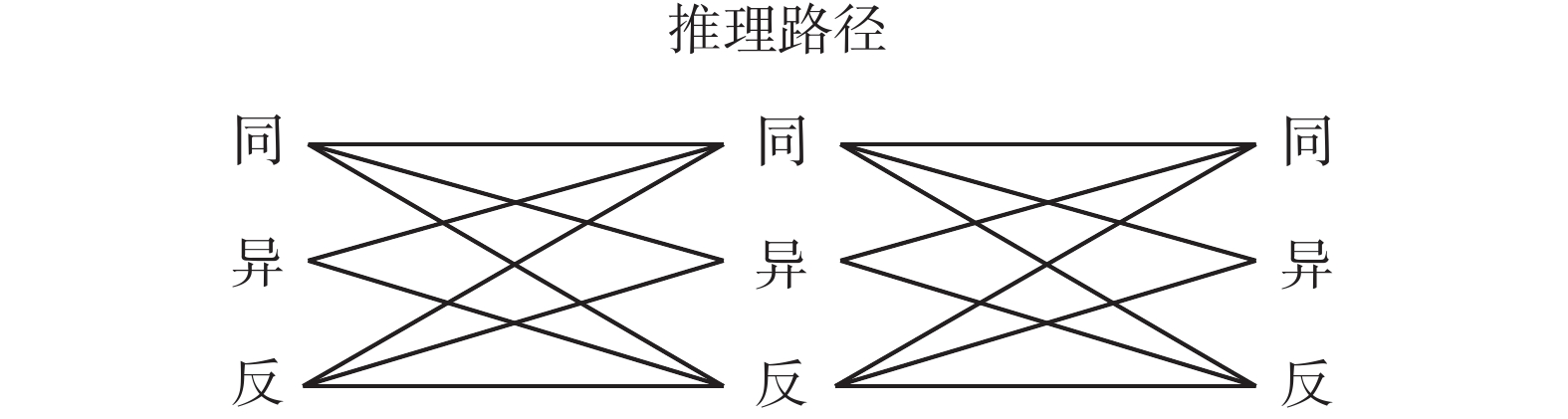Download: 图 3 同异反系统推理示意 Fig. 3 Reasoning of the same difference contradiction system

4.3 基于集对分析的推理应用实例

5 集对分析在智能决策中的应用 5.1 同异反智能决策

1) 作系统在正常情况下的决策；

2) 作系统在异常情况下的决策；

3) 作系统在反常情况下的决策；

4) 作系统在正常情况兼有异常情况下的决策；

5) 作系统在正常情况、异常情况、反常情况依次出现、交替出现、同时出现、随机出现情况下的决策；

6) 作系统在同异反不同情况下的综合决策；

7) 作系统在多种不确定性情况下的综合决策，具体结合各种不确定性因素的分析和联系数中i的不同取值展开讨论，评价决策风险；

8) 利用同异反数据作出评价系统潜在发展趋势的判断；

9) 利用同异反数据作评价系统显在发展趋势的判断；

10) 根据同异反数据确定的联系数的其他伴随函数进行决策等。

5.2 同异反智能决策的应用

6 集对分析在知识生态学中的应用 6.1 知识生态集对分析的原理

6.2 知识的同异反创新规律与同异反知识体系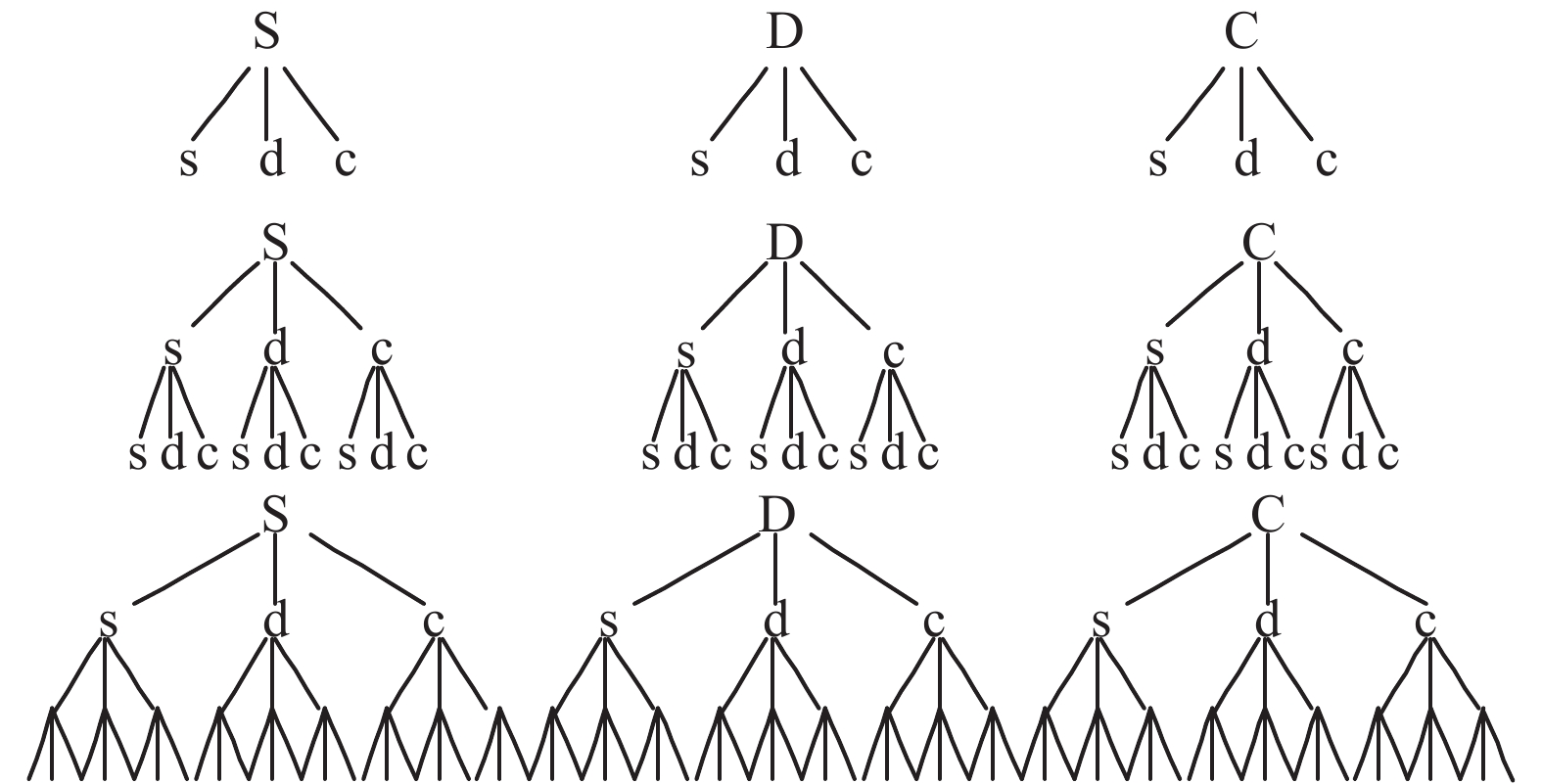Download: 图 4 同异反知识创新 Fig. 4 Innovation in the same difference contradiction knowledge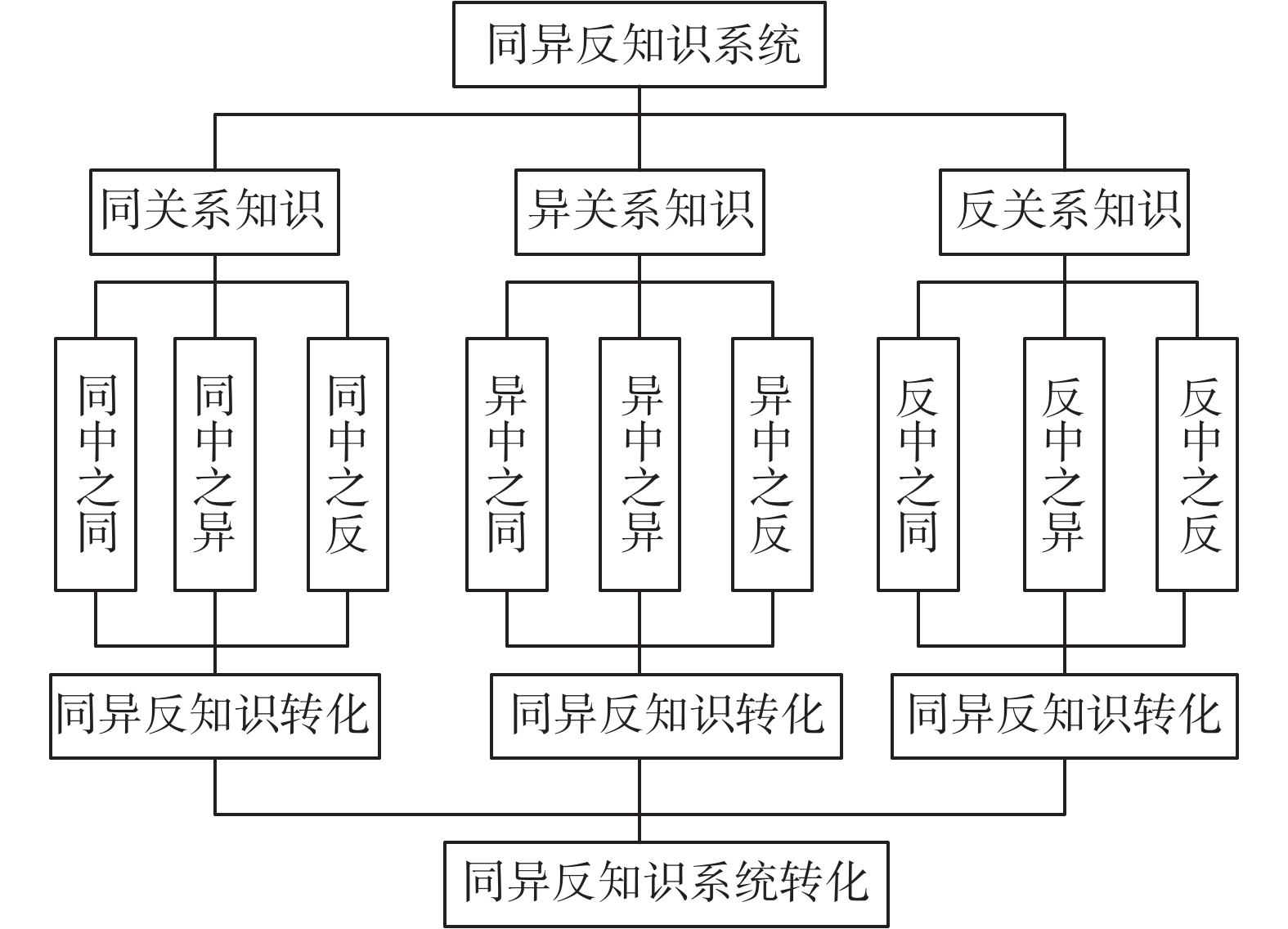Download: 图 5 同异反知识系统展开 Fig. 5 Expansion of the same difference contradiction system

6.3 应用实例

7 集对分析在自然语言和人类语言理解中的应用 7.1 自然语言和人类语言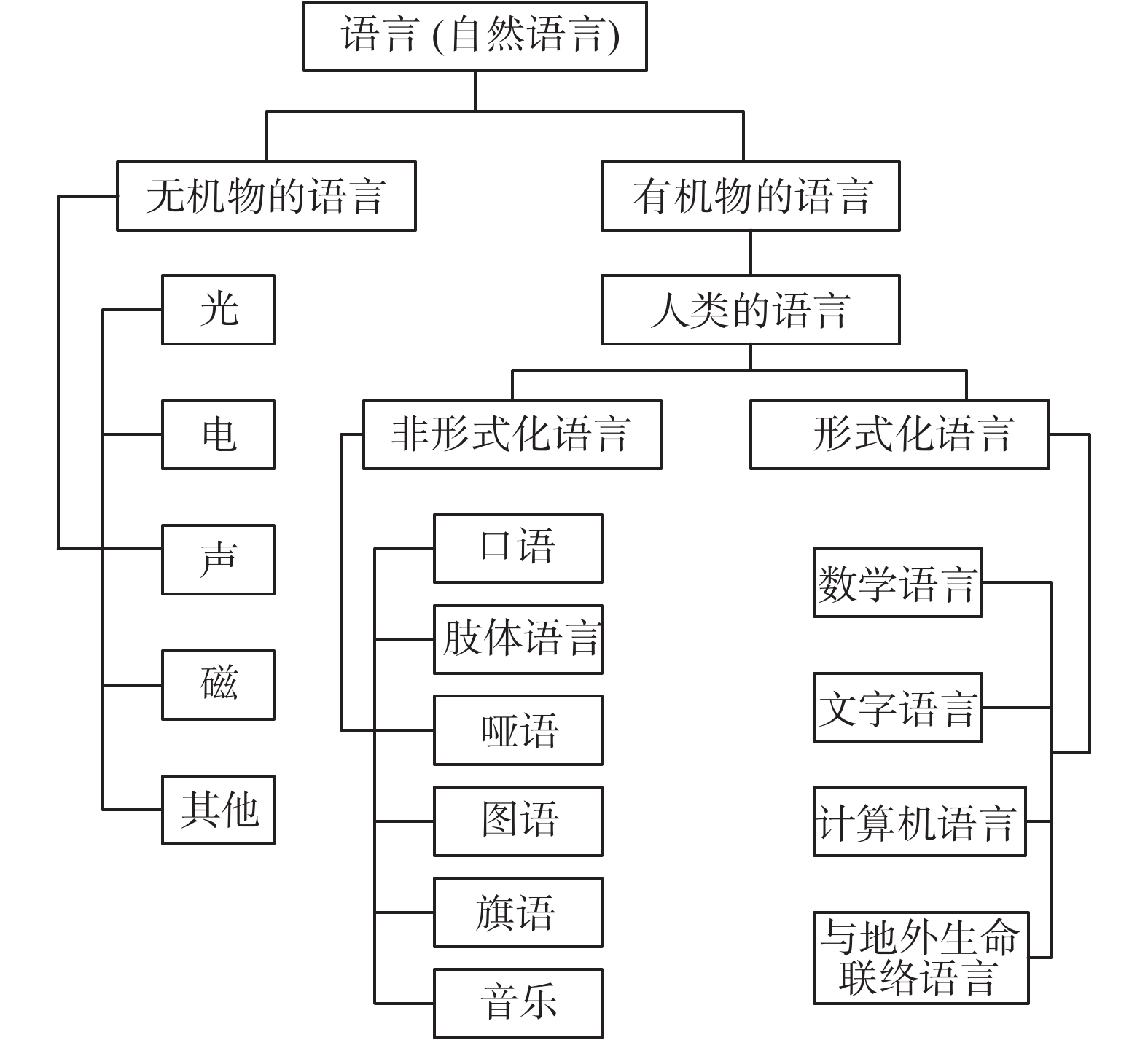Download: 图 6 自然语言的层次结构 Fig. 6 Natural of languages by hierarchical structure
7.2 人类语言理解和处理的集对分析

7.3 应用举例

1) 中文语义的相反相成

2) 中英文翻译

3) 语言的联系数刻画

8 集对分析在专家系统中的应用 8.1 专家系统应用集对分析的原理

8.2 集对分析在专家系统中的应用举例

9 集对分析与神经网络的融合及应用 9.1 融合原理与途径

9.2 应用举例

10 集对分析在智能工程中的应用 10.1 应用的必要性与可能性

10.2 应用举例

11 集对分析在智能社会中的应用 11.1 智能社会

11.2 应用举例

12 结束语

  赵克勤. 集对与集对分析——一个新的概念和一种新的系统分析方法[C]. 全国系统理论与区域规划研讨会. 包头, 中国, 1989: 8. (0)  赵克勤. 集对分析及其初步应用[M]. 杭州: 浙江科学技术出版社, 2000. (0)  王文圣, 李跃清, 金菊良, 等. 水文水资源集对分析[M]. 北京: 科学出版社, 2010. (0)  刘保相. 粗糙集对分析理论与决策模型[M]. 北京: 科学出版社, 2010. (0)  沈定珠. 体育用联系数学[M]. 北京: 中国教育文化出版社, 2007. (0)  郭瑞林. 作物育种同异理论与方法[M]. 北京: 中国农业科学技术出版社, 2011. (0)  赵克勤, 赵森烽. 奇妙的联系数[M]. 北京: 知识产权出版社, 2014. (0)  刘秀梅, 赵克勤. 区间数决策集对分析[M]. 北京: 科学出版社, 2014. (0)  蒋云良, 赵克勤, 刘以安, 等. 信息处理集对分析[M]. 北京: 清华大学出版社, 2015. (0)  王万军, 晏燕. 不确定信息处理的集对分析研究与应用[M]. 兰州: 兰州大学出版社, 2015. (0)  汪明武, 金菊良, 周玉良. 集对分析耦合方法与应用[M]. 北京: 科学出版社, 2014. (0)  潘争伟, 吴成国, 金菊良. 水资源系统评价与预测的集对分析方法[M]. 北京: 科学出版社, 2016. (0)  李丽红, 杨亚锋, 李言, 等. 集对推理与决策[M]. 北京: 清华大学出版社, 2016. (0)  汪明武, 金菊良. 联系数理论与应用[M]. 北京: 科学出版社, 2017. (0)  蒋云良, 赵克勤. 人工智能集对分析[M]. 北京: 科学出版社, 2017. (0)  赵克勤, 米红. 非传统安全与集对分析[M]. 北京: 知识产权出版社, 2010. (0)  蒋云良, 徐从富. 集对分析理论及其应用研究进展[J]. 计算机科学, 2006, 33(1): 205-209. JIANG Yunliang, XU Congfu. Advances in set pair analysis theory and its applications[J]. Computer science, 2006, 33(1): 205-209. DOI:10.3969/j.issn.1002-137X.2006.01.057 (0)  赵克勤. 集对分析的不确定性系统理论在AI中的应用[J]. 智能系统学报, 2006, 1(2): 16-25. ZHAO Keqin. The application of uncertainty systems theory of set pair analysis (SPU) in the artificial intelligence[J]. CAAI transactions on intelligent systems, 2006, 1(2): 16-25. (0)  赵克勤. 二元联系数A+Bi的理论基础与基本算法及在人工智能中的应用 [J]. 智能系统学报, 2008, 3(6): 476-486. ZHAO Keqin. The theoretical basis and basic algorithm of binary connection A+Bi and its application in AI [J]. CAAI transactions on intelligent systems, 2008, 3(6): 476-486. (0)  赵克勤. 成对原理及其在集对分析(SPA)中的作用与意义[J]. 大自然探索, 1998, 17(4): 90. ZHAO Keqin. The function and meaning of pair principle in the set pair analysis[J]. Exploeratioin nature, 1998, 17(4): 90. (0)  赵克勤. SPA的同异反系统理论在人工智能研究中的应用[J]. 智能系统学报, 2007, 2(5): 20-35. ZHAO Keqin. The application of SPA-based identical-discrepancy-contrary system theory in artificial intelligence research[J]. CAAI transactions on intelligent systems, 2007, 2(5): 20-35. (0)  赵克勤. 偏联系数[M]//中国人工智能学会. 中国人工智能进展. 北京: 北京邮电大学出版社, 2005: 884–885. (0)  赵克勤. 集对分析对不确定性的描述和处理[J]. 信息与控制, 1995, 24(3): 162-166. ZHAO Keqin. Disposal and description of uncertain ties based on the set pair analysis[J]. Information and control, 1995, 24(3): 162-166. (0)  赵克勤, 宣爱理. 集对论——一种新的不确定性理论方法与应用[J]. 系统工程, 1996, 14(1): 18-23, 72. ZHAO Keqin, XUAN Aili. Set pair theory——a new theory method of non—define and its applications[J]. Systems engineering, 1996, 14(1): 18-23, 72. DOI:10.3321/j.issn:1001-506X.1996.01.003 (0)  蔡自兴, 徐光佑. 人工智能及其应用[M]. 4版. 北京: 清华大学出版社, 2010. (0)  钟义信. 高等人工智能原理—观念•方法•模型•理论[M]. 北京: 科学出版社, 2014:1–452. (0)  朱其秀. 集对分析用于人工智能研究的思考[J]. 绍兴文理学院学报, 1996, 16(4): 113-118. ZHU Qixiu. Set pair annlysis for the study of artificial intelligence[J]. Journal of Shaoxing college of arts sciences, 1996, 16(4): 113-118. (0)  周志华, 曹存根. 神经网络及其应用[M]. 北京: 清华大学出版社, 2004. (0)  成科扬. 基于集对分析的指纹识别系统研究[M]//中国人工智能学会. 中国人工智能进展. 北京: 北京邮电大学出版社, 2005: 1475–1478. (0)  李德顺, 宫博, 许开立. 论金属矿山地质灾害模式识别模型[J]. 工业安全与环保, 2012, 38(3): 37-40. LI Deshun, GONG Bo, XU Kaili. Discussion on the geological disasters pattern recognition model of the metal mine[J]. Industrial safety and environmental protection, 2012, 38(3): 37-40. DOI:10.3969/j.issn.1001-425X.2012.03.013 (0)  高洁, 盛昭瀚. 集对分析聚类预测法及其应用[J]. 系统工程学报, 2002, 17(5): 458-462. GAO Jie, SHENG Zhaohan. Method and application of set pair analysis classified prediction[J]. Journal of systems engineering, 2002, 17(5): 458-462. DOI:10.3969/j.issn.1000-5781.2002.05.012 (0)  阎理, 阎滨. 相似系统集对分析[J]. 指挥技术学院学报, 2000, 11(3): 9-13. YAN Li, YAN Bin. The set pair analysis for similarity system[J]. Journal of institute of command and technology, 2000, 11(3): 9-13. (0)  刘秀梅, 赵克勤. 基于联系数的不确定空情意图识别[J]. 智能系统学报, 2012, 7(5): 450-456. LIU Xiumei, ZHAO Keqin. Inference method on intention with uncertain aerial information based on the connection number[J]. CAAI transactions on intelligent systems, 2012, 7(5): 450-456. DOI:10.3969/j.issn.1673-4785.201206016 (0)  张秀辉, 刘以安, 曹宁生, 等. 基于集对分析的雷达信号分选算法[J]. 现代雷达, 2010, 32(2): 35-37. ZHANG Xiuhui, LIU Yi'an, CAO Ningsheng, et al. Radar signal sorting method based on set pair analysis[J]. Modern radar, 2010, 32(2): 35-37. DOI:10.3969/j.issn.1004-7859.2010.02.008 (0)  LIU Yi’an, MONG Xianhai, WANG Shitong. Studies for image edge detection with set pair analysis[C]//DCABES. 2006: 373–76. (0)  赵森烽, 赵克勤. 概率联系数化的原理及其在概率推理中的应用[J]. 智能系统学报, 2012, 7(3): 200-205. ZHAO Senfeng, ZHAO Keqin. The principle of a connection number in probability and its application in probabilistic reasoning[J]. CAAI transactions on intelligent systems, 2012, 7(3): 200-205. DOI:10.3969/j.issn.1673-4785.201112014 (0)  蒋云良, 张裔智, 潘云鹤, 等. 基于集对分析的定量推理初探[J]. 计算机工程, 1996, 22(3): 286-290. JIANG Yunliang, ZHANG Yizhi, PAN Yunhe, et al. First exploration of quantitative inference of identity-discrepancy-contrary based on the set pair analysis[J]. Computer engineering, 1996, 22(3): 286-290. (0)  马守明, 王汝传, 叶宁. 基于集对分析的上下文感知不确定性的推理方法[J]. 南京邮电大学学报(自然科学版), 2009, 29(1): 64-67. MA Shouming, WANG Ruchuan, YE Ning. An uncertainty reasoning approach based on set pair analysis for context awareness[J]. Journal of Nanjing university of posts and telecommunications(natural science), 2009, 29(1): 64-67. DOI:10.3969/j.issn.1673-5439.2009.01.015 (0)  阎红灿, 王会芳, 马会霞. 基于集对分析的本体案例检索模型[J]. 微型机与应用, 2014, 33(21): 75-78. YAN Hongcan, WANG Huifang, MA Huixia. Ontology case retrieval model based on SPA[J]. Microcomputer and its applications, 2014, 33(21): 75-78. DOI:10.3969/j.issn.1674-7720.2014.21.025 (0)  赵克勤. 集对分析与同异反决策[J]. 决策探索, 1992(2): 14-15. ZHAO Keqin. The same differences counter-decision and Set pair analysis[J]. Policy research and exploration, 1992(2): 14-15. (0)  刘秀梅, 赵克勤. 基于联系数不确定性分析的区间数多属性决策[J]. 模糊系统与数学, 2010, 24(5): 141-148. LIU Xiumei, ZHAO Keqin. Multiple attributes decision-making of intervals based on analysis of the uncertainty of connection number[J]. Fuzzy systems and mathematics, 2010, 24(5): 141-148. (0)  赵森烽, 赵克勤. 联系概率的由来及其在风险决策中的应用[J]. 数学的实践与认识, 2013, 43(4): 165-171. ZHAO Senfeng, ZHAO Keqin. The contact probability in risk decision-making medium application[J]. Mathematics in practice and theory, 2013, 43(4): 165-171. DOI:10.3969/j.issn.1000-0984.2013.04.024 (0)  吴爱燕, 曾广平, 涂序彦. 集对云多属性群决策方法及应用[J]. 计算机科学, 2014, 41(12): 231-233, 250. WU Aiyan, ZENG Guangping, TU Xuyan. Study on set pair cloud multi-attribute group decision method[J]. Computer science, 2014, 41(12): 231-233, 250. DOI:10.11896/j.issn.1002-137X.2014.12.050 (0)  余国祥. 默会知识和显性知识的同异反集对分析[J]. 襄樊学院学报, 2008, 29(5): 84-88. YU Guoxiang. A SPA of tacit knowledge and explicit knowledge in identity, discrepancy and contrary[J]. Journal of Xiangfan university, 2008, 29(5): 84-88. DOI:10.3969/j.issn.1009-2854.2008.05.021 (0)  徐忆琳. 用SPA同异反系统理论研究知识创新规律[J]. 科学学研究, 2002, 20(3): 327-329. XU Yilin. Research on the law of knowledge innovation using IDC-SPA theory[J]. Studies in science of science, 2002, 20(3): 327-329. DOI:10.3969/j.issn.1003-2053.2002.03.022 (0)  杜春彦, 柴春红. 基于SPA的不确定推理模型[J]. 数学的实践与认识, 2004, 34(9): 130-134. DU Chunyan, CHAI Chunhong. Uncertain reasoning model based on set pair[J]. Mathematics in practice and theory, 2004, 34(9): 130-134. DOI:10.3969/j.issn.1000-0984.2004.09.022 (0)  张新华. 技术知识产权评估模型及应用[J]. 科研管理, 1999, 20(6): 47-51. ZHANG Xinhua. Evaluation model and application in technoque knowledge of righting[J]. Science research management, 1999, 20(6): 47-51. DOI:10.3969/j.issn.1000-2995.1999.06.007 (0)  周萍, 阎理. 集对分析与武器装备知识产权管理绩效评估[J]. 军事经济研究, 2005(6): 51-52. ZHOU Ping, YAN Li. Application of SPA to the performance evaluation of the intelligent property right management of the weapon equipment[J]. Militang economic research, 2005(6): 51-52. (0)  阮光册. 基于知识关联的检索结果聚类分析研究[J]. 情报科学, 2015, 33(2): 63-66. RUAN Guangce. Search result clustering analysis based on knowledge relation[J]. Information science, 2015, 33(2): 63-66. (0)  汪新凡. 基于联系数的纯语言多属性群决策方法[J]. 重庆工商大学学报( 自然科学版), 2006, 23(6): 580-584. WANG Xinfan. Pure linguistic information multi-attribute group decision-making method based on connection number[J]. Journal of Chongqing technology and business university(natural sciences edition), 2006, 23(6): 580-584. (0)  徐改丽. 基于语言评估和集对分析的多属性决策方法[J]. 重庆工学院学报(自然科学), 2009, 23(8): 85-89. XU Gaili. Multi-attribute decision-making method based on linguistic assessments and set pair analysis[J]. Journal of Chongqing institute of technology(natural science), 2009, 23(8): 85-89. (0)  栗飞, 刘博, 徐海峰. 基于集对分析的混合型多属性目标威胁评估[J]. 海军航空工程学院学报, 2012, 27(2): 219-222. LI Fei, LIU Bo, XU Haifeng. Threat assessment of hybrid multiple attribute targets based on set pair analysis[J]. Journal of naval aeronautical and astronautical university, 2012, 27(2): 219-222. (0)  徐峰. 猪病诊断专家系统研究[D]. 北京, 中国: 中国农业大学, 2005: 1–50. XU Feng. An expert system for pig diseases diagnosis[D]. Beijing, China: Agricultural University, 2005: 1–50. (0)  徐超. 进出口食品安全监管中风险评价和专家系统的研究[D]. 杭州, 中国: 浙江大学, 2013. XU Chao. The research of risk assessment and expert system for imported and exported food safety supervision[D]. Hangzhou, China: Zhejiang University, 2013. http://cdmd.cnki.com.cn/Article/CDMD-10335-1013185682.htm (0)  卞世晖. 专家系统中不确定性推理的研究与应用[D]. 合肥, 中国: 安徽大学, 2010. BIAN Shihui. Research and application of uncertainty reasoning in expert system[D]. Hefei, China: Anhui University, 2010. (0)  董卫华, 张政保. 数据挖掘及数据库技术在故障诊断专家系统中的应用[J]. 河北省科学院学报, 2006, 23(4): 20-23. DONG Weihua, ZHANG Zhengbao. Application of data mining and database technology in expert system for fault diagnosis[J]. Journal of the Hebei academy of sciences, 2006, 23(4): 20-23. DOI:10.3969/j.issn.1001-9383.2006.04.006 (0)  李佳, 王黎, 马光文, 等. 基于SPA-ANN耦合模型的年径流预测[J]. 水力发电学报, 2009, 28(1): 41-44. LI Jia, WANG Li, MA Guangwen, et al. Annual runoff forecasting based on SPA-ANN coupling model[J]. Journal of hydroelectric engineering, 2009, 28(1): 41-44. (0)  陈晶, 王文圣, 李跃清. 集对分析径向基函数神经网络预测模型[J]. 水文, 2011, 31(2): 11-14. CHEN Jing, WANG Wensheng, LI Yueqing. Prediction model of radial basis function neural network based on set pair analysis[J]. Journal of China hydrology, 2011, 31(2): 11-14. DOI:10.3969/j.issn.1000-0852.2011.02.003 (0)  赵晓慎, 吴海波, 陈丹. 集对分析在改进BP神经网络凌汛开河日期预测评估中的应用[J]. 水电能源科学, 2011, 29(12): 101-103. ZHAO Xiaoshen, WU Haibo, CHEN Dan. Study of set pair analysis in improving prediction and evaluation of BP neural network for breaking up date of ice flood[J]. Water resources and power, 2011, 29(12): 101-103. DOI:10.3969/j.issn.1000-7709.2011.12.029 (0)  魏邦友. 载人航天器综合测试数据评估方法的研究[J]. 电子质量, 2017(7): 28-30. WEI Bangyou. Research on evaluation method of comprehensive test data for manned spacecraft[J]. Electronics quality, 2017(7): 28-30. DOI:10.3969/j.issn.1003-0107.2017.07.008 (0)  黄德才, 张丽君, 赵克勤, 等. 基于a+bi型联系数的不确定网格静态调度算法 [J]. 计算机科学, 2007, 34(8): 126-129. HUANG Decai, GONG Weihua, ZHANG Lijun, et al. Grid task dynamic scheduling algorithm based on connective-number[J]. Computer engineering, 2007, 34(8): 126-129. DOI:10.3969/j.issn.1002-137X.2007.08.034 (0)  JIANG Yunliang, YANG Zhangxian, LI Gang, et al. A multi-sensor data fusion method based on the set-pair identity degree and connection number[C]//Proceedings of 2011 Workshop on Digital Media and Digital Content Management. Hangzhou, China, 2011: 298–301. (0)  王海颖, 刘以安, 薛松, 等. 基于集对分析联系度的多传感器数据融合方法[J]. 智能系统学报, 2017, 12(2): 1-8. WANG Haiying, LIU Yi’an, XUE Song, et al. Sensor data fusion based on connection degree of set pair analysis[J]. CAAI transactions on intelligent systems, 2017, 12(2): 1-8. (0)  李春磊, 莫蓉, 常智勇, 等. 综合考虑零件工艺设计经验和制造稳定性的工艺路线决策[J]. 计算机辅助设计与图形学学报, 2015, 27(12): 2385-2392. LI Chunlei, MO Rong, CHANG Zhiyong, et al. Decision-making of process route considering process planning experience and manufacturing stability[J]. Journal of computer-aided design and computer graphics, 2015, 27(12): 2385-2392. (0)  刘英, 陈志恒, 陈宇. 基于集对理论的砂轮主轴转动系统故障树分析[J]. 机械传动, 2014, 38(5): 77-80. LIU Ying, CHEN Zhiheng, CHEN Yu. Fault tree analysis of grinding wheel spindle rotation system based on set pair analysis theory[J]. Journal of mechanical transmission, 2014, 38(5): 77-80. (0)  卢凯, 荆彬彬, 吴焕, 等. 基于集对分析的干道绿波协调控制方案评价方法[J]. 东南大学学报(自然科学版), 2015, 45(3): 606-611. LU Kai, JING Binbin, WU Huan, et al. Evaluation method of arterial green wave coordinated control scheme based on set pair analysis[J]. Journal of southeast university(natural science edition), 2015, 45(3): 606-611. (0)  赵玉铃, 张廉. 集对分析联系数在黑启动vague集决策中的应用研究[J]. 智能系统学报, 2014, 9(5): 632-640. ZHAO Yuling, ZHANG Lian. Application of the ste-pair analyssi connection number in decision-making of black-start vague set[J]. CAAI transactions on intelligent systems, 2014, 9(5): 632-640. (0)  黄德才, 龚卫华, 张丽君, 等. 基于联系数的网格任务动态调度算法[J]. 计算机工程, 2009, 35(8): 112-115. HUANG Decai, GONG Weihua, ZHANG Lijun, et al. Grid task dynamic scheduling algorithm based on connective-number[J]. Computer engineering, 2009, 35(8): 112-115. DOI:10.3969/j.issn.1000-3428.2009.08.038 (0)  朱红宁, 张斌. 基于SPA的Web服务选取方法[J]. 计算机科学, 2009, 36(11): 32-35. ZHU Hongning, ZHANG Bin. Method of Web service selection based on SPA[J]. Computer science, 2009, 36(11): 32-35. DOI:10.3969/j.issn.1002-137X.2009.11.007 (0)  杨静, 李文平, 张健沛. 一种基于SPA的多数据流同异反分析法[J]. 武汉大学学报(信息科学版), 2011, 36(1): 92-97. YANG Jing, LI Wenping, ZHANG Jianpei. A data mining approach based on identical-different-contrary analysis[J]. Geomatics and information science of Wuhan university, 2011, 36(1): 92-97. (0)  张春英, 郭景峰. 集对社会网络α关系社区及动态挖掘算法 [J]. 计算机学报, 2013, 36(8): 1682-1692. ZHANG Chunying, GUO Jingfeng. The α relationship communities of set pair social networks and its dynamic mining algorithms [J]. Chinese journal of computers, 2013, 36(8): 1682-1692. (0)  陈晓, 郭景峰, 范超智. 基于联系度的主题关注网络社区发现方法研究[J]. 计算机工程与应用, 2017, 53(17): 85-93. CHEN Xiao, GUO Jingfeng, FAN Chaozhi. Community discovering based on connection degree in topic-attention network[J]. Computer engineering and applications, 2017, 53(17): 85-93. DOI:10.3778/j.issn.1002-8331.1608-0324 (0)  晏燕, 郝晓弘, 王万军. 一种隐私保护度量的集对分析方法[J]. 武汉大学学报(工学版), 2015, 48(6): 883-890. YAN Yan, HAO Xiaohong, WANG Wanjun. A set pair analysis method for privacy metric[J]. Journal of tsinghua university(science and technology), 2015, 48(6): 883-890. (0)